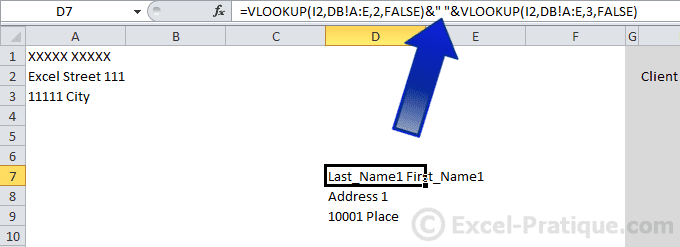A more recent version of the Excel course is available here: Excel Course

# Excel Course: Using a Database

The goal of this lesson is to provide you with an example of how to use data from a database.

In this example, our objective will be to enter a client's address into the bill using a drop-down list.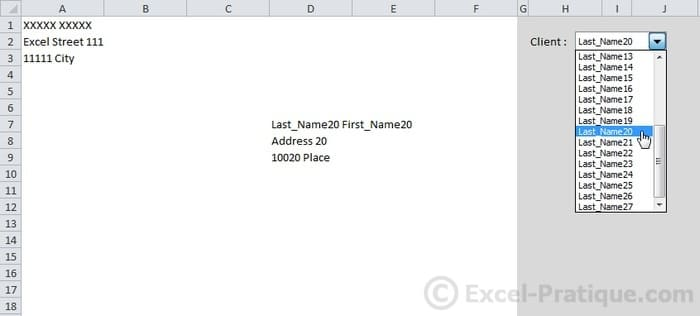We recommend that you do this exercise as you work through the following lesson: lesson10.xlsx

Start by inserting the VLOOKUP function at the location of the name and enter the required parameters (see image below):

Lookup_value: `I2`: the cell that contains the client number to search for.

Table_array: `DB!A:E`: select columns A to E of sheet "DB" (note: if you fill out the columns only, rather than the cells, you can enter your database later and you won't have to change any of the formulas).

Col_index_num: the column number entered here is the column number in Table_array. If we enter 2, we will get the name, 3, the first name, 4, the address, and 5, the city or town.

Range_lookup: `FALSE`: find an exact match.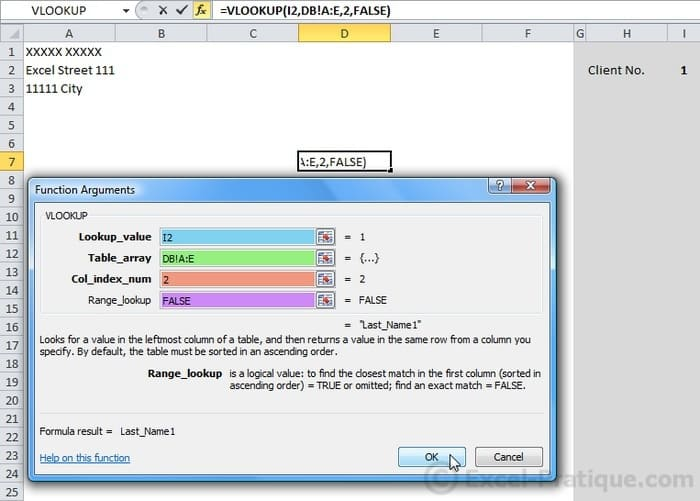The name of client number 1 is displayed.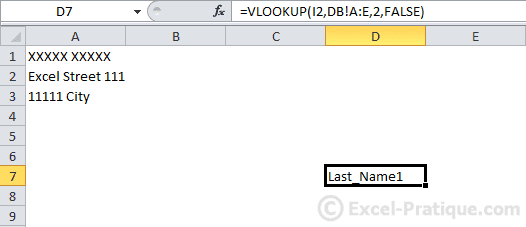Copy the formula to the following locations (without changing it):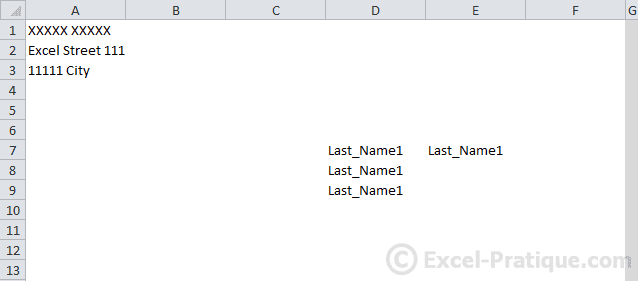Now edit the No_index_col parameter to get all the information.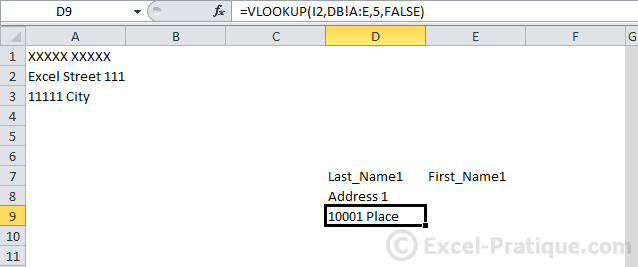## How to concatenate multiple values

In this intermediate example, A1 and B1 each contain a word. The goal is to return the two words in A2.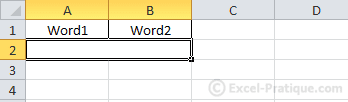To concatenate (= assemble) multiple values, use the `&` sign.

In this case, we would like to get: A1 + a space + B1, which gives us: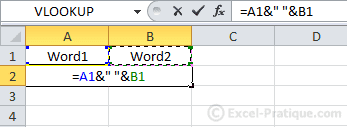The 2 words have been returned in A2 correctly and separated by a space.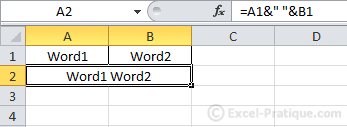Getting the last and first names on our bill, separated by a space, would be exactly the same with only one difference, which is that A1 and B1 would be replaced by formulas: